Difference between revisions of "Normal analytic space"

An analytic space the local rings of all points of which are normal, that is, are integrally-closed integral domains. A pointof an analytic spaceis said to be normal (one also says thatis normal at) if the local ring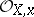is normal. In a neighbourhood of such a point the space has a reduced and irreducible model. Every simple (non-singular) point is normal. The simplest example of a normal analytic space is an analytic manifold.

In what follows the (complete non-discretely normed) ground fieldis assumed to be algebraically closed. In this case the most complete results on normal analytic spaces have been obtained (see ) and a normalization theory has been constructed  that gives a natural link between arbitrary reduced analytic spaces and normal analytic spaces. Let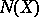be the set of points of an analytic spacethat are not normal and let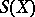be the set of singular points of(cf. Singular point). Then:

1)andare closed analytic subspaces of, and;

2) for,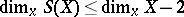(that is, a normal analytic space is smooth in codimension 1);

3) ifis a complete intersection atand if the above inequality holds, thenis normal at that point.

A normalization of a reduced analytic spaceis a pair, where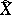is a normal analytic space and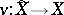is a finite surjective analytic mapping inducing an isomorphism of the open sets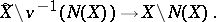The normalization is uniquely determined up to an isomorphism, that is, if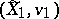and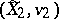are two normalizations,then there exists a unique analytic isomorphismsuch that the diagram commutes. The normalization exists and has the following properties. For every pointthe set of irreducible components ofatis in one-to-one correspondence with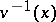. The fibre atof the direct image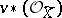of the structure sheaf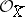is naturally isomorphic to the integral closure of the ringin its complete ring of fractions.

The concept of a normal analytic space overcan be introduced in terms of analytic continuation of holomorphic functions . Namely, a reduced complex space is normal if and only if Riemann's first theorem on the removal of singularities holds for it: Ifis an open subset andis a closed analytic subset not containing irreducible components of, then any function that is holomorphic on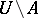and locally bounded onhas a unique analytic continuation to a holomorphic function on. For normal complex spaces Riemann's second theorem on the removal of singularities also holds: If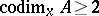at every point, then the analytic continuation in question is possible without the requirement that the function is bounded. A reduced complex spaceis normal if and only if for every open set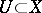the restriction mapping of holomorphic functionsis bijective. The property of being normal can also be phrased in the language of local cohomology — it is equivalent to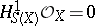(see ). For any reduced complex spaceone can define the sheaf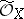of rings of germs of weakly holomorphic functions, that is, functions satisfying the conditions of Riemann's first theorem. It turns out that the ring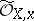is finite as an-module and equal to the integral closure ofin its complete ring of fractions. In other words,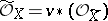, whereis the normalization mapping.

A normal complex space can also be characterized in the following manner: A complex space is normal if and only if every point of it has a neighbourhood that admits an analytic covering onto a domain of(see , ).

A reduced complex spaceis a Stein space if and only if its normalizationhas this property (see ). To normal complex spaces one can extend the concept of a Hodge metric (see Kähler metric). Kodaira's projective imbedding theorem  carries over to compact normal spaces with such a metric.

In algebraic geometry one examines analogues of normal analytic spaces: normal algebraic varieties (see Normal scheme). For algebraic varieties over a complete non-discretely normed field the two concepts are the same (see , ).

How to Cite This Entry:
Normal analytic space. Encyclopedia of Mathematics. URL: http://encyclopediaofmath.org/index.php?title=Normal_analytic_space&oldid=18341
This article was adapted from an original article by D.N. Akhiezer (originator), which appeared in Encyclopedia of Mathematics - ISBN 1402006098. See original article